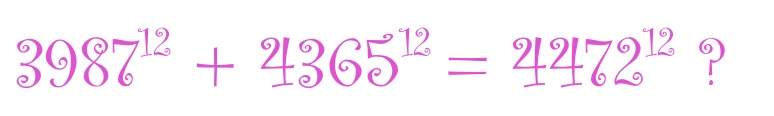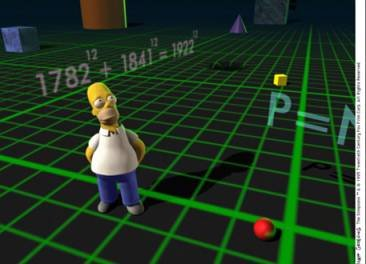# Near misses in Fermat’s last theorem

Posted by: Gary Ernest Davis on: March 2, 2011Fermat’s last theorem, proved by Andrew Wiles, states that there are no positive whole number solutions for$a, b, c$ to the equality$a^n+b^n=c^n$ for$n\geq 3$

Yet in an episode of The Simpson’s it was noted that$3987^{12} + 4365^{12} = 4472^{12}$, apparently contradicting Fermat’s last theorem.Of course this is not a contradiction because$3987^{12} + 4365^{12}$ is not actually equal to$4472^{12}$.

So why did The Simpson’s episode say these two numbers were equal?

Well,$3987^{12} + 4365^{12} =63976656349698612616236230953154487896987106$

while$4472^{12} = 63976656348486725806862358322168575784124416$

So, starting from the left we see that the$11^{th}$ digit in$3987^{12} + 4365^{12}$ is a 9, while for$4472^{12}$ it is an 8.

“What is the best way to measure the “closeness” of a near-solution to a^p+b^p=c^p? E.g. how “close” is 3987^12 + 4365^12 = 4472^12 really?”
x
The left most digits of these large numbers represent very large quantities.
x
Maybe one way to compare these two numbers is to look at their ratio$\frac{3987^{12} + 4365^{12}}{4472^{12}}$.
x
If we have a calculator that’s accurate to 10 decimal places we get the result
x$\frac{3987^{12} + 4365^{12}}{4472^{12}} =1.0000000000$
x
So to 10 decimal places the ratio$\frac{3987^{12} + 4365^{12}}{4472^{12}}$ is 1.
x
It’s not until we get a more accurate calculator that we see, in fact,
x$\frac{3987^{12} + 4365^{12}}{4472^{12}} \approx 1.0000000000189426406$
x
Another way to think about the relationship between$3987^{12} + 4365^{12}$ and$4472^{12}$ is to take the twelfth root of$3987^{12} + 4365^{12}$.
x
If we really had equality between$3987^{12} + 4365^{12}$ and$4472^{12}$ then$(3987^{12} + 4365^{12})^{1/12}$ would be$4472$.
x
If we have a calculator that’s accurate to 12 digits, we get$(3987^{12} + 4365^{12})^{1/12}= 4472.00000000$
x
It’s only with a more accurate calculator that we see$(3987^{12} + 4365^{12})^{1/12}\approx 4472.0000000070592907$
x
This raises a sort of interesting question:
x
Given a “small” potential error$\epsilon$ and an integer$n\geq 3$, what solutions are there to
x$a^n+b^n=(c+\epsilon)^n$
x
for positive integers$a, b, c$ ?

### 3 Responses to "Near misses in Fermat’s last theorem"You can find thousands of near-misses by solving the following equation:
A^n+B^n = C^n + !
or A^n+B^n = C^n – !
The difference is always !. I tested these up to n = 1000 and these still hold.
Surely based on asymptotic argument, these are counter-examples to FLT?38305^3 + 51762^3 = 57978^3
49193^3 + 50920^3 = 63086^3I think I found a set of formulas which work to find the series of best “near solutions” to Fermat’s Last Theorem out to infinity. I can’t be sure … just have an ordinary PC and Lotus spreadsheet which is accurate
only to 18 digits. Anyone who would like to see the formulas can email me … I’ll attach my final spreadsheet, which does work for 55 equations in a row … I’m sure of that. HH